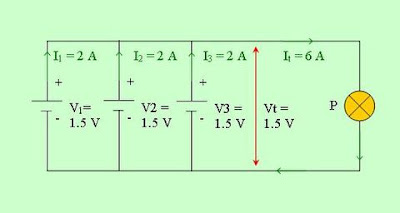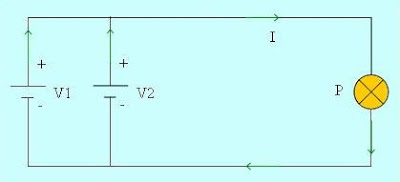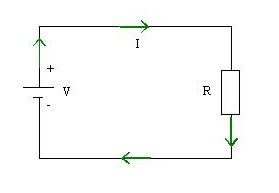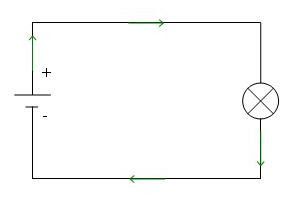Calculation of parallel connection

In a closed circuit connected in parallel, the amount of voltage at any place in the circuit is the same. While the amount of current flow and the amount of resistance can vary. To determine the amount of voltage and the amount of electrical resistance in parallel circuit, can use the formula:

It = I1 + I2 + I3 + • • • • • • + In
1/Rt = 1/R1 + 1/R2 + 1/R3 + • • • • • • + 1/Rn
Vt = V1 = V2 = V3 = • • • • • • = Vn

Where:
- Vt = number of voltage
- Rt = total resistance
- It = number of flow
- V1, V2, V3 = voltage of each source
- R1, R2, R3 = resistance of each component
- I1, I2, I3 = flow through each channel
- Vn = voltage at the source n
- Rn = resistance at the n components
- In = current in the channel n

Sample question:
What is the amount of voltage and current generated in the installation of three parallel battery voltage and current are respectively 1.5 V and 2 A?
Completion:
It = I1 + I2 + I3 = 2 + 2 + 2 = 6 A
Vt = V1 = V2 = V3 = 1.5 V
The number of voltage generated is a fixed 1.5 V and current of 6 ACalculation of the series connection

In a closed circuit connected in series, the current flow in any place in the circuit is the same. While the amount of voltage and the amount of resistance can vary. To determine the amount of voltage and the amount of electrical resistance in the series, can use the formula:

Vt = V1 + V2 + V3 + • • • • • • + Vn
Rt = R1 + R2 + R3 + • • • • • • + Rn
It = I1 = I2 = I3 = • • • • • • = In

Where:
- Vt = number of voltage
- Rt = total resistance
- It = number of flow
- V1, V2, V3 = voltage of each source
- R1, R2, R3 = resistance of each component
- I1, I2, I3 = flow through each channel
- Vn = voltage at the source n
- Rn = resistance at the n components
- In = current in the channel n

Sample question 1:
What is the amount of voltage and current generated in the installation of a series of three battery voltage and current are respectively 1.5 V and 1 A?
Completion:
Vt = V1 + V2 + V3 = 1.5 + 1.5 + 1.5 = 4.5 VOLTS
It = I1 = I2 = I3 = 1 A
The amount of voltage generated 4.5 VOLTS and the current is fixed at 1 AExample question 2:
What is the amount of electrical resistance produced in the installation of a series of three electric load value of 4 Ω, 6 Ω, 8 Ω?
Completion:
Rt = R1 + R2 + R3 = 4 + 6 + 8 = 18 Ω
The number of generated electrical resistance of 18 Ω

Based on the above, it can be concluded that:
1. Amount of voltage from a power source connected to the series is the sum of each voltage
2. Amount of resistance from a number of electrical loads or electrical components are connected series is the sum of each resistance
3. Currents in a series circuit is the same in all parts of the circuit

Parallel connection

In electrical circuits, we recognize two types of standard connection, namely: the series connection and parallel connection. When the encounter is the form of connection other than that, basically a variation of the series and parallel connections. Previous articles have discussed the series and will now discuss the parallel connections.

Parallel connection
If several sources, load, or electrical devices connected in the same two pins, called a parallel connection. Figure 1 shows the parallel connection of three batteries.Figure 1. Parallel connection of three batteries

Unlike the series connection, although one of the three removable battery, will not disturb the circuit in parallel connection, the lamp will remain lit.Figure 2. One of the three removable battery, will not disturb the circuit in parallel connection

Series connection

In electrical circuits, we recognize two types of standard connections, namely: the series connection and parallel connection. When the encounter is the form of connection other than that, basically a variation of the series and parallel connections. Here are described the series connection.

Series connection
If several sources, load, or electrical devices connected in a row with each other, so that current flows in relay started from the first, second, third and so on, called the series connection. Figure 1 shows the series connection of three batteries.Figure 1. Series connection of three batteries

In a series connection, each of which carries a conductor of electric current to another part. Therefore, if the three batteries are connected in series with the lamp, one battery is disconnected, then cut off the electric current in series connection, so that the lamp will not light.Figure 2. Cut off the electric current in series connection

Value conversion unit

Other units in the form of greater numbers, sometimes inconvenient when we write it. For such cases, use the value of the conversion units such as the table below.

The following units commonly used in electrical engineering subjects
 Value unit Epsilon Symbols Equation Tera 1012 T 1 THz=1000000000000 Hz Giga 109 G 1 GHz=1000000000 Hz Mega 106 M 1 MV=1000000 V kilo 103 k 1 kV= 1000 V milli 10-3 m 1 mA= 0.001 A micro 10-6 µ 1 µA= 0.000001 A nano 10-9 n 1 nF= 0.000000001 F pico 10-12 p 1 pF= 0.000000000001 F femto 10-15 f 1 fF=0.000000000000001 F

How to pay electrical energy?

The amount of electrical energy used in a home is measured by using an electric meter (kWh meter in general term in Indonesia), which is connected to PLN's power supply lines. Calculate the electric meter electric energy usage in kWh units. Electricity bills charged to the user based on the amount of electrical energy consumption. The costs listed in the bill is calculated by using the formula:

Cost of electricity = electricity energy x cost per unit usage

Sample questions:
A flood warning light works on power 500 W. This lamp is lit every night for 10 hours. Electrical energy consumption cost is Rp 100 per kWh. What is the cost that must be paid for one month (30 days)?

Completion:
Cost of electricity = (500 W x 10 hours x 30) x Rp 100 = (0.5 kW x 300 hours) x Rp 100 = Rp 15000
The total cost to be paid amounting to Rp 15000

The amount of energy

A number of electric power can generate power or energy. With electricity we can get a light, sound, heat, movement, and others. The amount of energy produced by an electrical device depends on:
- Rating power devices
- Long working time device

The greater the power the device, the more the amount of energy produced in a certain period. The longer the device worked, the more the amount of energy produced at a given power rating. Thus, it can be concluded that:

Electrical energy (W) = Power (P) x time (t)

The quantity of electrical energy is written with the notation W, and electrical energy unit called kilo Watt hour notation written in letters kWh.

Sample questions:
A water heater with 800 W power, takes 2 hours to boil water in a tank. What is the amount of energy released in this process?

Completion:
W = P x t = 800 W x 2 hours = 0.8 kW x 2 hours = 1.6 kWh
The amount of energy expenditure of 1.6 kWh

Electric power

In incandescent lamps, electric power converted into the form of light and heat energy. If a light on in an hour, so long as the lamp uses a certain amount of energy. When the lights were on for two hours, the lamp uses energy twice as much from the one hour.

From the description above, can be concluded:
The amount of energy used is proportional to the time the lights burning.
Scale electric power notation is written with the letter P, and electric power units called Watt a letter written with the notation W.

In electrical circuits, power is proportional to the voltage and current. The greater the flow and the greater the voltage, the greater the power generated. This statement can be written with the formula:Where:
- P indicates electrical power to the unit Watt (W)
- V shows the line voltage to the units of volts (V)
- I show the units of electric current Ampere (A)

Sample question 1:
An electric iron works with 220 V voltage and current flowing in it for 3 A, what power it produces?

Completion:
P = V x I = 220 V x 3 A = 660 W
The resulting power of 660 W

Sample question 2:
See picture below. If known lamp power (P) of 12 W, voltage (V) of 12 V, what is the electrical current (I) is flowing?Completion:
I = P / V = 12 W / 12 V = 1 A
The electric current flowing at 1 A

Electrical resistance and Ohm's law

Electrical resistance (R) is the friction or obstacles that are given a substance to an electrical current. With this friction or obstacles, causing the motion of electrons decreases. Barriers that hinder electron movement is called the resistance, while the instrument is called a resistor or resistance. Unit to measure electrical resistance is called Ohm or by the symbol Ω (read Omega).

The greater the resistance of a conductor, the smaller the electrical current flowing to him.

Ohm's law
The relationship between electric current, voltage, and electrical resistance in a circuit expressed in Ohm's lawWhere:
- V shows the line voltage to the units of volts (V)
- I shows the electrical current to the units of Ampere (A)
- R indicates a unit of electrical resistance Ohm (Ω)

Sample questions:
See the picture below. Given the value of electric current (I) for 6 Ampere, and the value of resistance (R) of 15 Ω. What is the required voltage (V)?Completion:
V = I x R = 6 x 15 = 90 Volts

Voltage electricity

Electric voltage (V) is the force that moves electric current to flow along an electrical circuit. According to the theory of electron, a positively charged object if the object lack negatively charged electrons and if it is the excess electron. In different circumstances this is the emergence of the energy charge potential which lies between these objects. Therefore, if a piece of conducting wire connected between the two, there will be a transfer of energy, and goes on as long as there are different voltages. Voltage difference due to the occurrence of each charge has a potential power to move a load in a way attractive or reject.

Voltage difference between two points can be generated by conducting put pressure on electricity from a power plant, battery, or voltage sources other.Batteries as a source of electric voltage

Unit to measure the electric voltage is called the Volt, or abbreviated with the letter V. PLN source voltage is 220 V, battery voltage typically 1.5 V, while the voltage batteries are usually 6 V and 12 V.

Electrons flow

According to the theory of electron: electrons flow from the excess electrons to the electron-deficient or from the negative charge toward the positive charge. But according to the direction of the current agreement (not the direction of flow of electrons) always moves from the positive to the negative. Look at the pictures belowFigure Flow Theory Based Electricity Electrons: electrons flow from negative to positiveFigure Flow Based Electricity Agreement: electrons flow from positive to negative